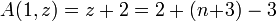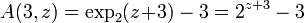# Ackermann function

In computability theory, the Ackermann function or Ackermann-Péter function is a simple example of a computable function that is not primitive recursive. The set of primitive recursive functions is a subset of the set of general recursive functions. Ackermann's function is an example that shows that the former is a strict subset of the latter. .

## Definition

The Ackermann function is defined recursively for non-negative integers m and n as follows::$A(m, n) = \begin{cases} n+1 & \mbox{if } m = 0 \\ A(m-1, 1) & \mbox{if } m > 0 \mbox{ and } n = 0 \\ A(m-1, A(m, n-1)) & \mbox{if } m > 0 \mbox{ and } n > 0. \end{cases}$

## Rapid growth

Its value grows rapidly; even for small inputs, for example A(4,2) contains 19,729 decimal digits .

## Holomorphic extensions

The lowest Ackermann functions allow the extension to the complex values of the second argument. In particular,

A(0,z) = z + 1$A(1,z) =z+2=2+(n\!+\!3)-3$$A(2,z) =2z+3=2\!\cdot\!(n\!+\!3)-3$

The 3th Ackermann function is related to the exponential on base 2 through$A(3,z) = \exp_2(z\!+\!3)-3=2^{z+3}-3$

The 4th Ackermann function is related to tetration on base 2 through

A(4,z) = tet2(z + 3) − 3

which allows its holomorphic extension for the complex values of the second argument. 

For n > 4 no holomorphic extension of A(n,z) to complex values of z is yet reported.